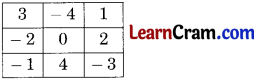# DAV Class 6 Maths Chapter 3 Brain Teasers Solutions

The DAV Class 6 Maths Book Solutions Pdf and DAV Class 6 Maths Chapter 3 Brain Teasers Solutions of Integers offer comprehensive answers to textbook questions.

## DAV Class 6 Maths Ch 3 Brain Teasers Solutions

Question 1A.
(a) The number of integers between (- 10) and 3 is ______
(i) 11
(ii) 13
(iii) 12
(iv) 14
Solution:
(iii) 12
– 10, – 9, – 8, – 7, – 6, – 5, – 4, – 3, – 2, – 1, 0, 1, 2, 3
which are twelve (12) in numbers.
Hence, (iii) is the correct option.

(b) If we subtract (- 10) from (- 11) we get
(i) – 1
(ii) 1
(iii) – 21
(iv) 21
Solution:
(i) – 1
(-11) – (- 10)
= – 11 + 10
= – 1
Hence, (i) is the correct option.

(c) Square of 2 subtracted from cube of (- 1) is
(i) 3
(ii) 5
(iii) – 5
(iv) 1
Solution:
(iii) – 5
(- 1)3 – (2)2
= – 1 – 4
= – 5
Hence, (iii) is the correct option.

(d) Value of | -7 | + (- 6) + [3| is
(i) – 10
(ii) 4
(iii) 10
(iv) – 4
Solution:
(ii) 4
7 – 6 + 3 = 10 – 6 = 4
Hence, (ii) is the correct option.

(e) Which of the following does not lie to the right side of (- 61) on the number line?
(i) – 10
(ii) 18
(iii) – 49
(iv) – 73
Solution:
(iv) – 73
– 73 < – 61,
so – 73 lie on the left side of the – 61.
Hence (iv) is the correct option.(a) Write any two integers less than (- 101).
Solution:
– 105, – 200

(b) Find the value of |(- 30) – (- 7)|.
Solution:
|(- 30) – (- 7)| = |- 30 + 7|
= |- 23|
= 23

(c) Which integer added to (- 4) will give the integer 5?
Solution:
(- 4) + x = 5
x = 5 – (- 4)
or x = 5 + 4 .
∴ x = 9

(d) Simplify and write its opposite (- 3) × 5 × (- 1).
Solution:
(- 3) × 5 × (- 1)
= (- 3) × (- 1) × 5
= 3 × 5 = 15
Opposite of 15 is – 15.

(e) Find the sum of the greatest negative integer and smallest positive integer.
Solution:
Greatest negative integer = – 1
Smallest positive integer = + 1
Sum = – 1 + (+ 1) = 0Question 2.
Indicate using integers.

(a) 200 BC
Solution:
200 BC = – 200

(b) 5° Celsius below zero
Solution:
5° Celsius below zero = – 5

(c) Win by 3 goals
Solution:
Win by 3 goals = + 3

(d) 40 km above sea level.
Solution:
40 km above sea level = + 40

Question 3.
Write the opposite of the following statements.

(a) India won the match by 3 wickets.
Solution:
India lost the match by 3 wickets.

(b) Mohan withdrew ₹ 2500 from his bank account.
Solution:
Mohan deposited ₹ 2500 to his bank account.

Question 4.
Write any three integers which are

(a) Smaller than – 25
Solution:
– 26, – 27, – 28

(b) Greater than – 191
Solution:
– 190, – 189, – 188Question 5.
Arrange is ascending order:
– 104, 48, – 69, 13, – 7, – 96, – 48, 5
Solution:
Ascender of the given integers is
– 104, – 96, – 69, – 48, – 7, 5, 13, 48

Question 6.
Find the value on the number line.

(a) (- 3) + 5 – 7
Solution: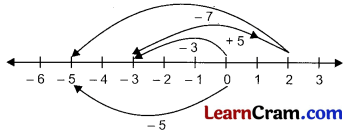Hence, – 3 + 5 – 7 = – 5

(b) 8 + (- 6) + (- 2)
Solution: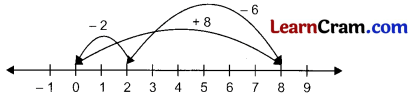Hence, 8 + (- 6) + (- 2) = 0Question 7.
Simplify:

(a) (- 400) + 781 + (- 1400) + (- 81) + 300
Solution:
(- 400) + 781 + (- 1400) + (- 81) + 300
= 781 + 300 – 400 – 1400 – 81
= 1081 – 1881
= – 800

(b) (- 273) + (- 541) + 900 + (- 511)
Solution:
(- 273) + (- 541) + 900 + (- 511)
= – 273 – 541 – 511 + 900
= – 1325 + 900
= – 425

Question 8.
Subtract:

(a) – 9 from 0
Solution:
Subtract – 9 from 0 = 0 – (- 9)
= 0 + 9
= 9

(b) – 115 from – 115
Solution:
Subtract – 115 from – 115 = – 115 – (- 115)
= – 115 + 115
= 0

Question 9.
Find the value of:

(а) (- 6) × [9 + (- 11)]
Solution:
(- 6) × [9 + (- 11)]
= (- 6) × [9 – 11]
= (- 6) × (- 2)
= 12

(b) 325 × (- 641) + 325 × (- 359)
Solution:
325 × (- 641) + 325 × (- 359)
= 325 × [(- 641) + (- 359)]
= 325 × (- 1000)
= – 325000

(c) 52 × (- 1)19 × (- 2)3 × 32 × (- 10)3
Solution:
52 × (- 1)19 × (- 2)3 × 32 × (- 10)3
= 25 × (- 1) × (- 8) × 9 × (- 1000)
= 200 × (- 9000)
= – 1800000Question 10.
Compare.
18 × (- 3) + 21 and 18 × [(- 3) + 21]
Solution:
18 × (- 3) + 21 = – 54 + 21 = – 33
18 × [(- 3) + 21] = 18 × 18 = 324
∴ – 33 < 324.

Question 11.
Fill in the blanks.

(a) There are ____ integers from – 4 to 11.
Solution:
16

(b) Natural numbers are called ______ integers (Positive/Negative).
Solution:
Positive

(c) The additive inverse of 6 is ____.
Solution:
– 6

(d) 168 + ___ = 0
Solution:
– 168

(e) The predecessor of – 249 is _____.
Solution:
– 250

(f) [(- 2) + (- 7)] × 3 = 3 × ___ + 3 × ____
Solution:
– 2, – 7

(g) The opposite of (- 3) × 2 × (- 1) is _____
Solution:

(h) All the negative integers are ______ than zero.
Solution:
less

(i) 14, 7, 0, – 7, _____, ______
Solution:
– 14, – 21Question 12.
Write ‘True’ or ‘False’ for the following statements.

(a) The absolute value of an integer is always greater than integer.
Solution:
False

(b) The product of 9 negative integers is positive.
Solution:
False

(c) Cube of 11 has 1 in its units place.
Solution:
True

(d) The base in 73 is 3.
Solution:
False

(e) 38 ÷ 35 = 33
Solution:
TrueQuestion 13.
Fill in the missing places with proper integers.

(a)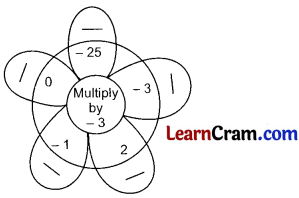Solution:(b)Solution: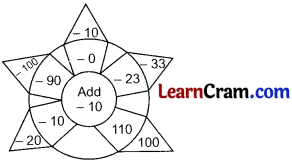### DAV Class 6 Maths Chapter 3 HOTS

Question 1.

(a) Calculate
1 – 2 + 3 – 4 + 5 – 6 + …. + 179 – 180.
Solution: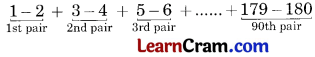Work in pair, we get
(- 1) + (- 1) + (- 1) + (- 1)
As total terms are 180, so when we work in pairs, total terms become half of 180, i.e. 90.
∴ (- 1) + (- 1) …. up to 90 terms
(- 1) × 90 = – 90

(b) Find the value of 5 + (- 5 ) + 5 + (- 5) + 5 ……….. if the number fives are-
(i) 148
(ii) 191
Solution:
(i) As 148 is an even number, so when we work in pair we get
5 + (- 5) + 5 + (- 5) + 5 + (- 5)
In this way 5 and – 5 get cancelled out with each other in all the pairs formed,
so we get 0 + 0 + …….. which is equal to 0.

(ii) As 191 is an odd number, so when we work in pairs we get
5 + (- 5) + 5 + (- 5) + 5 + (- 5) …………. 5,
In this way 5 and – 5 get cancelled out with other except the last 5.
So we get 0 + 0 + …………… 5 = 5

Question 2.
A cement company gains ₹ 12 per bag of white cement sold and gets a loss of ₹ 8 per bag of grey cement sold.

(a) If the company sells 3500 bags of white cement and 5000 bags of grey cement in a month, find the gain or loss.
Solution:
Gain on sale of 1 bag of white cement = ₹ 12
∴ Gain on sale of 3500 bags of white cement = ₹ 12 × 3500 = ₹ 42,000
Loss on sale of 1 bag of grey cement = ₹ 8
∴ Loss on sale of 5000 bags of grey cement = ₹ 8 × 5000 = ₹ 40,000
As, gain > loss, so there is a gain overall.
∴ Gain = 42,000 – 40,000 = ₹ 2,000

(b) If the number of grey cement bags sold is 6000, how many bags of white cement should the company sell to have neither gain or loss?
Solution:
Let number of white bags sold = n
For neither gain nor loss, we have 6000 × 8 = n × 12
∴ n = $$\frac{6000 \times 8}{12}$$
= 4000 bagsQuestion 1.
(a) 148 ÷ 146 = 14
(b) $$\left(\frac{2}{3}\right)^{\cdots} \div\left(\frac{2}{3}\right)^9=\frac{1}{\left(\frac{2}{3}\right)^3}$$
(c) 65 × 63 × 62 = 6
(d) (23)6 = 2….
Solution:
(a) 148 ÷ 146
= 148 – 6
= 142
Hence, 2 is to be filled in the blank.

(b) $$\left(\frac{2}{3}\right)^{\cdots} \div\left(\frac{2}{3}\right)^9=\frac{1}{\left(\frac{2}{3}\right)^3}$$
⇒ $$\left(\frac{2}{3}\right)^{\cdots} \div\left(\frac{2}{3}\right)^9=\frac{1}{\left(\begin{array}{l} 2 \\ 3 \end{array}\right)^3}$$
⇒ $$\left(\frac{2}{3}\right)^{\cdots} \div\left(\frac{2}{3}\right)^9=\left(\frac{2}{3}\right)^{-3}$$
⇒ $$\left(\frac{2}{3}\right)^{\cdots}=\left(\frac{2}{3}\right)^9 \times\left(\frac{2}{3}\right)^{-3}$$
⇒ $$\left(\frac{2}{3}\right)^{\cdots}=\left(\frac{2}{3}\right)^9$$
⇒ $$\left(\frac{2}{3}\right)^{\cdots}=\left(\frac{2}{3}\right)^6$$
Hence, 6 is to be filled in the blank,

(c) 65 × 63 × 62 = 6……….
= 65 + 3 + 2
= 6……
= 610
= 6…..
Hence, 10 is to be filled in the blank.

(d) (23)6 = 2….
⇒ 23 × 6 = 2….
⇒ 218 = 2….
Hence, 18 is to be filled in the blank.Question 2.
Which of the following statements are true?
(a) (4)8 ÷ (4)7 = (4)2
(b) $$\left(\frac{2}{3}\right)^6 \times\left(\frac{2}{3}\right)^0=\left(\frac{2}{3}\right)^6$$
(c) $$\left(\frac{1}{4}\right)^4 \div\left(\frac{1}{4}\right)^{10}=\left(\frac{1}{4}\right)^6$$
(d) $$\frac{2}{3}+\left(\frac{1}{2} \div \frac{1}{3}\right)=\frac{2}{3} \div\left(\frac{1}{2}+\frac{1}{3}\right)$$
Solution:
(a) (4)8 ÷ (4)7 = 48-7
= 4
∴ 4 ≠ (4)2
Hence, it is not true.

(b) $$\left(\frac{2}{3}\right)^6 \times\left(\frac{2}{3}\right)^0=\left(\frac{2}{3}\right)^6$$
L.H.S = $$\left(\frac{2}{3}\right)^6 \times\left(\frac{2}{3}\right)^0$$
= $$\left(\frac{2}{3}\right)^{6+0}$$
= $$\left(\frac{2}{3}\right)^{6}$$
= R.H.S
∴ $$\left(\frac{2}{3}\right)^{6}$$ = $$\left(\frac{2}{3}\right)^{6}$$
Hence, it is true.

(c) $$\left(\frac{1}{4}\right)^4 \div\left(\frac{1}{4}\right)^{10}=\left(\frac{1}{4}\right)^6$$
L.H.S = $$\left(\frac{1}{4}\right)^4 \div\left(\frac{1}{4}\right)^{10}$$
= $$=\left(\frac{1}{4}\right)^{4-10}$$
= $$\left(\frac{1}{4}\right)^{-6}$$
∴ $$\left(\frac{1}{4}\right)^{-6}$$ ≠ $$\left(\frac{1}{4}\right)^{6}$$
Hence, it is not true.

(d) $$\frac{2}{3}+\left(\frac{1}{2} \div \frac{1}{3}\right)=\frac{2}{3} \div\left(\frac{1}{2}+\frac{1}{3}\right)$$
L.H.S:
$$\frac{2}{3}+\left(\frac{1}{2} \times \frac{3}{1}\right)$$
= $$\frac{2}{3}+\frac{3}{2}$$
= $$\frac{4+9}{6}$$
= $$\frac{13}{6}$$

R.H.S.
$$\frac{2}{3} \div\left(\frac{1}{2}+\frac{1}{3}\right)$$
= $$\frac{2}{3} \div\left(\frac{3+2}{6}\right)$$
= $$\frac{2}{3} \div \frac{5}{6}$$
= $$\frac{2}{3} \times \frac{6}{5}$$
= $$\frac{4}{5}$$
∴ $$\frac{13}{6}$$ ≠ $$\frac{4}{5}$$
Hence, it is not true.Question 3.
Simplify:
(a) 24 + 32
(b) 23 – 22
(c) (- 1)16 + (- 1)2 + (- 1)– 18
(d) (- 5)6 ÷ (- 5)2
(e) 18th power of 3 ÷ 15th power of 3
Soution:
(a) 24 + 32
= 16 + 9 = 25

(b) 23 – 22
= 8 – 4 = 4

(c) (- 1)16 + (- 1)2 + (- 1)– 18
= 1+ 1 + 1 = 3

(d) (- 5)6 ÷ (- 5)2
= (- 5)4

(e) 18th power of 3 ÷ 15th power of 3
= (3)18 (3)15
= (3)18 – 15
= (3)3
= 27

Question 4.
Write the base and exponents of the following :
(a) 26
Solution:
26, Base 2 and exponent 6.

(b) 30
Solution:
30, Base 3 and exponent 0.

(c) (- 1)6
Solution:
(- 1)6, Base (- 1) and exponent 6.

(d) (8)– 6
Solution:
(8)– 6, Base 8 and exponent – 6.

(e) (6)– 8
Solution:
(6)– 8, Base 6 and exponent – 8.Question 5.
Express the following as a rational number with positive exponent :
(a) $$\left(\frac{3}{2}\right)^{-4}$$
(b) (3– 3)2
(c) (83 ÷ 85) × 8– 4
(d) $$\left(\frac{2}{3}\right)^{-3} \times\left(\frac{2}{3}\right)^5$$
Soution:
(a) $$\left(\frac{3}{2}\right)^{-4}$$
= $$\frac{1}{\left(\frac{3}{2}\right)^4}$$
= $$\left(\frac{2}{3}\right)^4$$

(b) (3– 3)2
= 3– 3 × 2
= 3– 6
= $$\left(\frac{1}{3}\right)^6$$

(c) (83 ÷ 85) × 8– 4
= 83 – 5 × 8– 4
= 8– 2 × 8– 4
= 8– 2 – 4
= 8– 6
= $$\left(\frac{1}{8}\right)^6$$

(d) $$\left(\frac{2}{3}\right)^{-3} \times\left(\frac{2}{3}\right)^5$$
= $$\left(\frac{2}{3}\right)^{-3+5}$$
= $$\left(\frac{2}{3}\right)^2$$

Question 6.
Calculate: 1 – 2 + 3 – 4 + 5 – 6 + … + 79 – 80
Solution:
1 – 2 + 3 – 4 + 5 – 6 + … + 79 – 80
= (1 + 3 + 5 + 7 + … + 79) – (2 + 4 + 6 + 8 + … 80)
= 1600 – 1640
= – 40Question 7.
Find the value of: 5 + (- 5) + 5 + (- 5) + 5 + … if the number of 5 are
(a) 148
(b) 91
Solution:
(a) 5 + (- 5) + 5 + (- 5) + 5
Number of 5 is 148 (even)
∴ Number of 5 is 74 and Number of (- 5) is 74
∴ 5 × 74 + (- 5) × 74
= 370 – 370
= 0

(b) 5 + (- 5) + 5 + (- 5) + 5 …
Number of 5 is 91 (odd)
Let us see if number of 5 is 90
∴ Number of 5 is 45 and Number of (- 5) is 45
∴ 5 × 45 + (- 5) × 45
= 225 – 225 = 0
The value of 91th 5 = 5
∴ Total value = 0 + 5 = 5Question 8.
Complete the magic square.Solution: